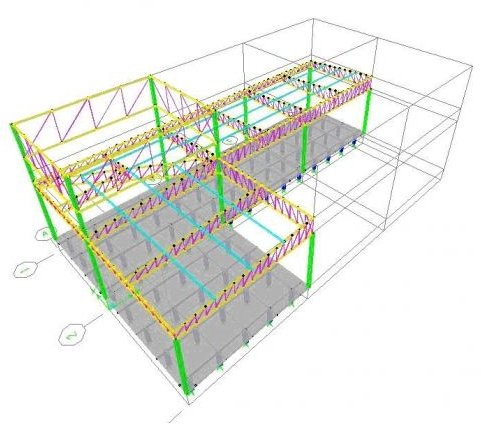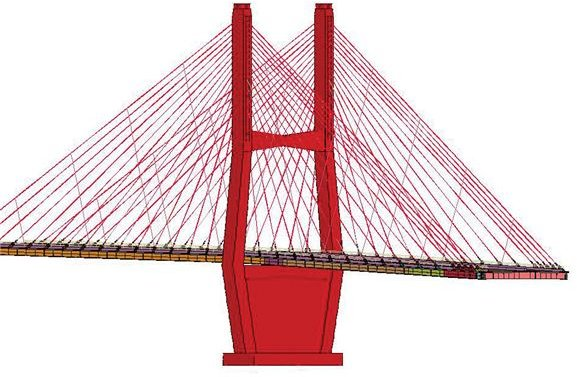# Basics of Finite Element Analysis

## History

Since the middle of the 20th century, intricate issues in civil, aeronautical, and nuclear engineering necessitated the development of finite element analysis. An extension of integral calculus, finite element analysis computes stress points, loads, and other fundamental physical characteristics based on mathematical equations. By the end of the 20th century, the field of finite element analysis had developed into an important design tool that has radically improved the engineering designs for numerous industrial applications.

## Mechanics Of Finite Element Analysis

Finite element analysis is performed by dividing the structure into a considerable number of small elements that could be of variable dimensions and that could possess different characteristics depending on their location within the structure. The physical properties of the elements that need to be examined could be numerous depending upon the application, such as the coefficient of thermal expansion, shear modulus, density, and heat transfer. Through the subdivision of the structure into small elements, the structural problems are altered into a finite group of equations that can be conveniently solved by the use of powerful computers. Finite element analysis being a numerical technique, it determine the solutions of partial differential equations and integral equations by either complete elimination of the partial differential equations or their description into ordinary differential equations.

## Advantages Of Finite Element Analysis

Finite element analysis provides a comprehensive indication of the distribution of stresses and a visualization of the bending or twisting of structures where a failure may occur. Predicting failure due to the development of stresses has become simpler, by an accurate indication of the affected location, thus allowing the designers to redesign accordingly. Finite element analysis ensures savings of enormous amounts that would be necessary if failure is identified only after the manufacture of products or completion of structural projects. The initial prototype designs are improved by using finite element analysis as a tool that has decreased the time required to move from the conceptual stage to actual production. Due to the advancement of the finite element analysis, designs can be created, improved, and finalized before the actual production or commencement of a project. Thus, the advantages of finite element analysis include superior design with an accurate analysis of the critical design parameters, increased production, and reduced failures.

## Applications of Finite Element Analysis

The finite element analysis is applied in numerous engineering disciplines, including car manufacturing. An accurate prediction of chassis damage by accident is made possible by simulating structural chassis performance under varying car speeds and angles of accident. The chassis is made of multiple composites, and car components are attached by inserts, with the aim to achieve a smooth and flat chassis. A variety of tests are simulated by the use of finite element analysis to achieve the required safety standards. Similarly, finite element analysis is being utilized in the design of airport pavements, considering the types of aircrafts with different number of wheels, and also determines the characteristics necessary for the construction materials. The finite element analysis can be used to determine the methods to improve the beam strength of bridges, and thus increase the life cycle of bridges.